X Close

## dB Frequency Ohms Law

Sound is vibrating air, which our ears can hear
The number of vibrations per second is called   (cps)   'cycles per second'
(cps)   is changed to Hz   in honour of the physicist Hertz.

Sound frequency measurement is 20 Hz - 20k Hz   (20 - 20,000 Hz)
Human hearing is approx 30 Hz - 16k Hz   and most sensitive between 2k - 3k Hz

The lowest note on a double bass or bass guitar is open E   (42Hz)
The highest notes depending on the instrument is approx   2k Hz
Above these highest notes are the harmonics.

#### Musical   Notation

Notation is based on 12 notes, between each doubling of the frequency (octave)
Between (A 110 Hz - A 220 Hz) are 12 notes
Between (A 220 Hz - A 440 Hz) are 12 notes   and so on

The division between the notes is based on the 12th root of 2
Each single adjacent note is called a Semi-tone.
Each second alternate note is called a Tone.

8 (Octave) of the 12 notes can be used to make a scale, as 2 tetra-chords
Eg.   (tone, tone, tone, semi-tone)   (tone, tone, tone, semi-tone)
octave is also used to describe double or half the frequency   (ratio 1:2)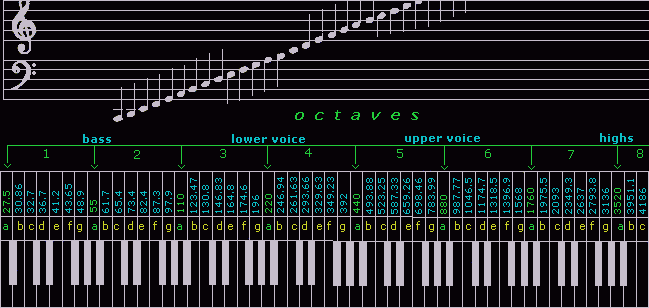#### 3.32 octaves   =   1 decade

Audio engineering   uses the octave (frequency ratio 1:2) for frequency measurement.   Other areas of engineering, use the decade (ratio 1:10).   3.32 octaves is 1 decade.   There is a small difference between 3 octaves and 3.32 octaves.   The 0.32 is sometimes omitted in the text for simplicity.

#### What is dB?

D deci is one tenth.   B Bell   in honour of Alexander Graham Bell
dB is a ratio for power (1:10)   therefore:

0dB       is   1 with   0 zero     (1)
10dB     is   1 with   1 zero     (10)
20dB     is   1 with   2 zeros   (100)
60dB     is   1 with   6 zeros   (1,000,000)
120dB   is   1 with   12 zeros (1,000,000,000,000)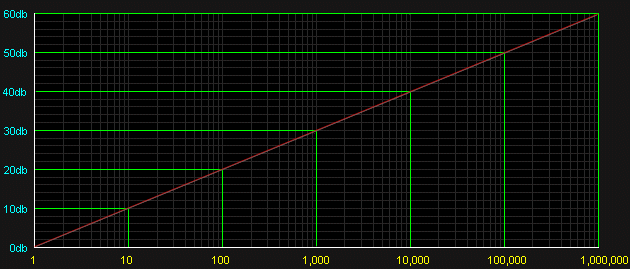Logarithms   are a simple and literal way of writing large numbers.
Hundreds of years ago, writing was done with a quill (ducks feather) dipped in ink.
Doing math with large numbers was time consuming and painful for ducks.
1,000,000   has 6 zeros   and can be abbreviated as   (106)   or   (60dB)

Using   0dB   from a reference point         B   is followed by letters   0dBm   or   0dBSPL   etc

#### How to use dB

1dB   (10√10)   is a number,   when x multiplied by itself 10 times   = 10

1dB   (1.261)     1.26
2dB   (1.262)     1.26 x 1.26   = 1.59
3dB   (1.263)     1.26 x 1.26 x 1.26   = 2

4dB   (1.264)     1.26 x 1.26 x 1.26 x 1.26   = 2.52
5dB   (1.265)     1.26 x 1.26 x 1.26 x 1.26 x 1.26   = 3.18
6dB   (1.266)     1.26 x 1.26 x 1.26 x 1.26 x 1.26 x 1.26   = 4

7dB   (1.267)     1.26 x 1.26 x 1.26 x 1.26 x 1.26 x 1.26 x 1.26   = 5
8dB   (1.268)     1.26 x 1.26 x 1.26 x 1.26 x 1.26 x 1.26 x 1.26 x 1.26   = 6.3
9dB   (1.269)     1.26 x 1.26 x 1.26 x 1.26 x 1.26 x 1.26 x 1.26 x 1.26 x 1.26   = 8
10dB (1.2610)   1.26 x 1.26 x 1.26 x 1.26 x 1.26 x 1.26 x 1.26 x 1.26 x 1.26 x 1.26   = 10

3dB steps   Most use of dB is rounded off in 3dB steps   as 3dB to 6dB then to 10dB
dB 1 2 4 5 7 8 9 dB are not often used   except by academics doing pure math.

1dB   =   x 1.26 power change   we hear as  smallest  loudness difference (laboratory only).
1dB       sound change is too small and not used in most calculations (laboratory only).
9dB       is not used because the 1dB difference to 10dB is too small to hear.

8dB   is rounded to     6dB or 10dB
9dB   is rounded to   10dB
14dB   is rounded to   13dB
22dB   is rounded to   23dB
29dB   is rounded to   30dB
32dB   is rounded to   33dB
47dB   is rounded to   46dB

23dB   (1.2623)       is     1.26 x by itself 23 times   =   200
46dB   (1.2646)       is     1.26 x by itself 46 times   =   40,000

(1.26x) is too awkard.   It is simpler to do it as in the examples below.

16dB   is     (10dB is 10)            (6dB is 4)            10 x 4   =   40
23dB   is     (20dB is 100)          (3dB is 2)          100 x 2   =   200
46dB   is     (40dB is 10,000)     (6dB is 4)    10,000 x 4   =   40,000
53dB   is     (50dB is 100,000)   (3dB is 2)  100,000 x 2   =   200,000

With pensil and paper continue to practice.   No need for calculator.
All dB rounded to nearest 3dB step.   3dB   6dB   then to next   10dB.

6dB / octave steps   are used for specifications of speaker crossovers and EQ filters.
Each   (6dB / octave)   step is described as an 'order'.     (rarely used above 24dB)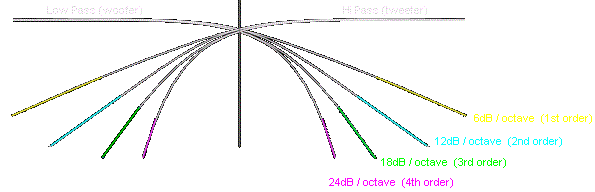6dB / octave   (1st order)
12dB / octave   (2nd order)
18dB / octave   (3rd order)
24dB / octave   (4th order)

6dB   is   4
12dB   is   4 x 4   =   16
18dB   is   4 x 4 x 4   =   64
24dB   is   4 x 4 x 4 x 4   =   256

#### Understanding dB for sound

Our ears expand when it is quiet to hear detail   and   contract when it is loud.

1dB   =   x 1.26 power change   we hear as smallest loudness difference (laboratory only).
1dB       sound change is too small and not used in most calculations (laboratory only).
9dB       is not used because the 1dB difference to 10dB is too small to hear.

3dB   =   x 2     power change     we only hear as a bit louder.
6dB   =   x 4     power change     we only hear as a bit louder again.
10dB =   x 10   power change     we only hear as double as loud.

Audible difference
Difference between   100 -   200 Watt sound system,   is minimal   (3dB)
Difference between   100 - 1,000 Watt sound system,   is twice as loud   (10dB)

#### 0dB SPL   (Sound Pressure Level)

Threshold of hearing, smallest modulation of atmospheric pressure.
0dB SPL   (Sound Pressure Level)   0.0002 dynes/cm²   (20micro Pascals) at 2kHz

80 - 100dB SPL   loud to VERY LOUD
60 -   80dB SPL   loud sound needing to speak loudly to be heard.
40 -   60dB SPL   average noise level of the world we live and work in.
20 -   40dB SPL   quiet ambient background, will allow 60dB dynamic range.
0 -   20dB SPL    threshold of hearing,   dependant on frequency.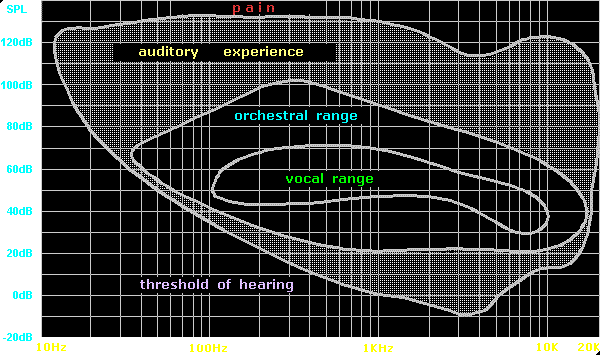0dB SPL   (Sound Pressure Level)   0.0002 dynes/cm²   (20micro Pascals) at 2kHz
Reference for,   threshold of hearing the smallest modulation, of atmospheric pressure.

By paying attention to this graph, it can be seen that at the threshold of hearing, our ears are approx 60dB (one million times) more sensitive at 2kHz than at 40Hz.   This is the reason a loudness switch is put on most domestic sound equiptment.   The loudness switch boosts the bass to compensate for our hearing at low level.   But at high power, all frequencies tend to be heard at approx the same level.

After the telephone was invented, it was noticed that a ten times power change (10dB) was only heard as doubble or half as loud.   A two times power change (3dB) is noticeable.   Later, these measurements became called the Fletcher-Munson curves.   The large energy variations in our inviroment (light, sound, touch, taste, smell, temperature etc) are compressed by our sensory system, in a subjective and complex mannar.

The sound.whsites.net site also contains a similar overview with precise figurers.
www.sound.whsites.net

#### Hearing damage

Hearing damage   is specified from 84 dB SPL+ for 4hrs of continuous industrial machine noise.
Time is halved for each 3dB increase (87dB/2hrs) (90dB/1hr) etc.   These figures vary slightly from diferent sources.

A false belief of many young people is that excessive loud music is not harmfull.   Reverberation in venues can hold sound at a continuous level, adding 20-30dB more sound energy, similar to machine noise.   The distortion of large sound systems exaggerate the problem.   Popular music is often excessively compressed within a 10dB dynamic range.   Many so called recording engineers aim to achieve 0dB dynamic range.   Excessive use of compression to exaggerate loudness is irrisponsible, similar to continuous machine noise.   Excessive compression also causes music to sound flat and lifeless.

Music can be played at high levels, with less auditory fatigue.   However, the music must be fully dynamic with little to no compression (peak limiting only to avoid amplifier clipping) and played through 4 way active, low distortion sound systems.   Venues must be acoustically treated and non reverberant, similar to a jungle.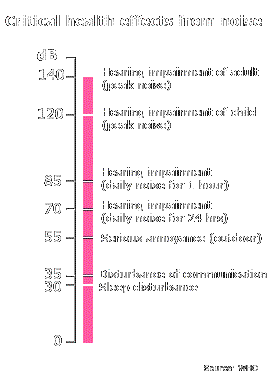The following statments and pic are from the Rockwool insulation web site.   www.rockwool.com

What is a good sound environment ?

Noise should be dampened to such an extent that it no longer interferes with the activity you were set out to do. Just 30 dB(A) is disturbing to sleep. Noise with sound levels of 35 dB(A) or more interferes with the intelligibility of speech in smaller rooms.

Even lower background levels are needed for adequate speech intelligibility for vulnerable groups - such as the hearing impaired, the elderly, children in the process of language and reading development, and individuals who are not familiar with the spoken language.

Rooms with many hard surfaces may result in disturbing echo which must be avoided. A reverberation time below 0.6 seconds is desirable, even in a quiet environment.

A 10 dB difference is perceived by the human ear as a doubling (or halving) of the audible sound.

#### Other applications for dB

Reference for electrical signal levels etc.   dB is followed by another letter
(m for milli-Watt)   (u for 775mV)   (V for Volt)   (W for Watt)   etc.

0dBm   =   1 milli-Watt   (600Ω at 775mV)
0dBu   =   775 mV
0dBV   =   1 Volt
0dBW   =   1 Watt

#### Frequency   Wavelength   Time

Depending on temperature, humidity, distance above sea level.

Sound is air vibrating, traveling as a wave motion at approx 344 meters per second.
Wavelength is the distance of one complete wave cycle.

Wavelength   (Symbol Greek letter Lambda)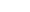=   344 / frequency.

344 / 42Hz   =   8.2 meter
344 / 1kHz   =   0.344 meter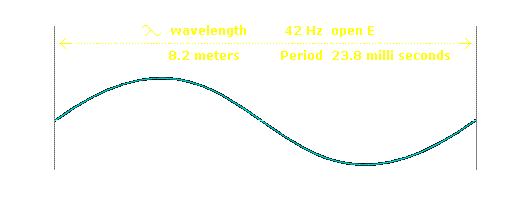Frequency Hz   (cycles per sec)           100Hz         1kHz         10kHz
Wavelength   is distance of 1 cycle       3.44m         0.344m      34.4mm
Time   is the period for 1 cycle               10milliS       1milliS       100microS
Power   is height of wave (amplitude)

Below middle C   262 Hz   hearing becomes omni-directional.

1 Acoustic Watt   at 1 meter, radiating spherically from a point source = 109dB SPL

Inverse square law   As direct sound doubles in distance, energy decreases to 1/4 (-6dB).

Critical Distance   is the point where direct and reverberant sound energy is equal.

RT60   is measurement of time; reverberation decreases to one millionth (-60dB).

Echo   is sound reflected back from 10 meters or more, heard as distinct repeat.

Acoustical Masking 6dB   any sound of 6dB+ masks others of similar frequency.

Spectral Energy of Music   is flat to approx 1k Hz, then approx -6dB per octave.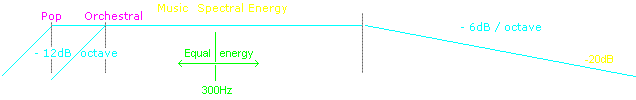#### Ohms Law

Electro-magnetic energy is a mystery.

Ohms Law are the rules applying to our relationship to electricity, adapted from water flow.

Low Resistance   is   Large Diameter Wire,   or   Water Pipe.

High Resistance   is   small diameter wire,   or   water pipe.

Ampere   Amount of electricity,   or water flow,   is,
a function of,   Pressure (Volt)   and   (Ohm) Resistance

```
/V\
/A|R\
```

Watt   Power   =   Pressure (Volt)   x   (Ampere) Amount.

``` /W\
/V|A\
```

Ω Ohm or R Resistance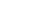is constant Resistance over time or frequency.

Z Impedance   is variable Resistance over time or frequency.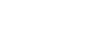F Capacitance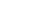refers to storage of electricity, as an electrostatic charge.   (Z decreases with frequency)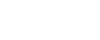L Inductance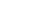refers to a coil of wire inducing magnetism as storage of energy in a magnetic field.   (Z increases with frequency)

• R or Ω   measured in Ohms
is constant Resistance over frequency
• Z Impedance   measured in Ohms
is variable Resistance over frequency, with any combinations of (R - XL - XC)
• XC Capacitive Reactance   measured in micro-Farads (uF)
is variable Impedance over frequency. (Amperes leads Volts 90deg)
• XL Inductive Reactance   measured in milli-Henry (mH)
is variable Impedance over frequency. (Volts leads Amperes 90deg)

D.C.   Direct Current   (constant polarity over time)

A.C.   Alternating Current   (changes polarity over time)

R.M.S.   Root Mean Square:   is the A.C. equivalent to D.C. as resultant energy.   Driving in traffic changing direction (A.C.) as an equivalent to driving constantly in one direction. (D.C.)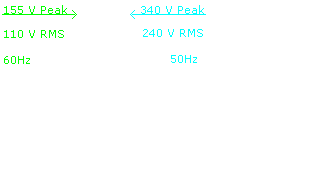110 Volt   (R.M.S.)   60Hz   A.C. mains power
maximum peak of sine wave 155 Volt.

240 Volt   (R.M.S.)   50Hz   A.C. mains power
maximum peak of sine wave 340 Volt.

End of Topic 1

Created: 24-Oct-2009